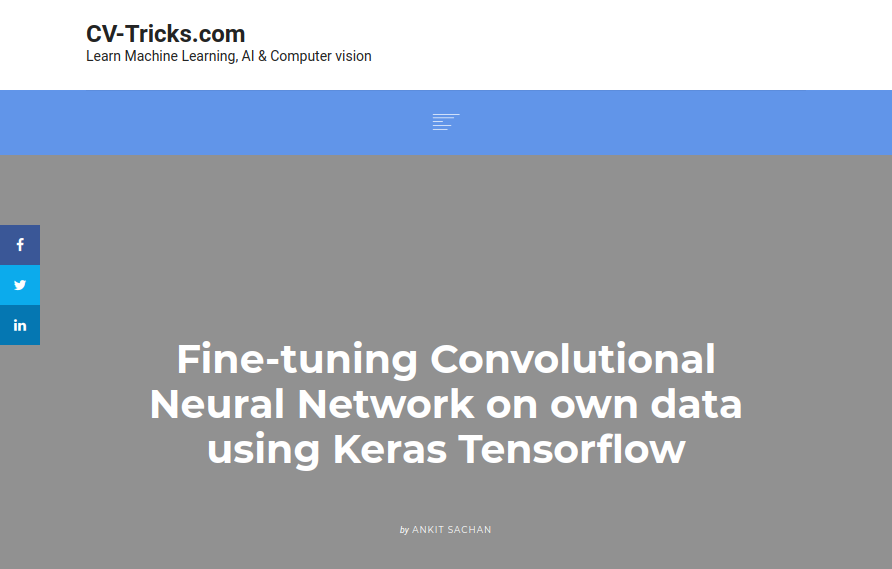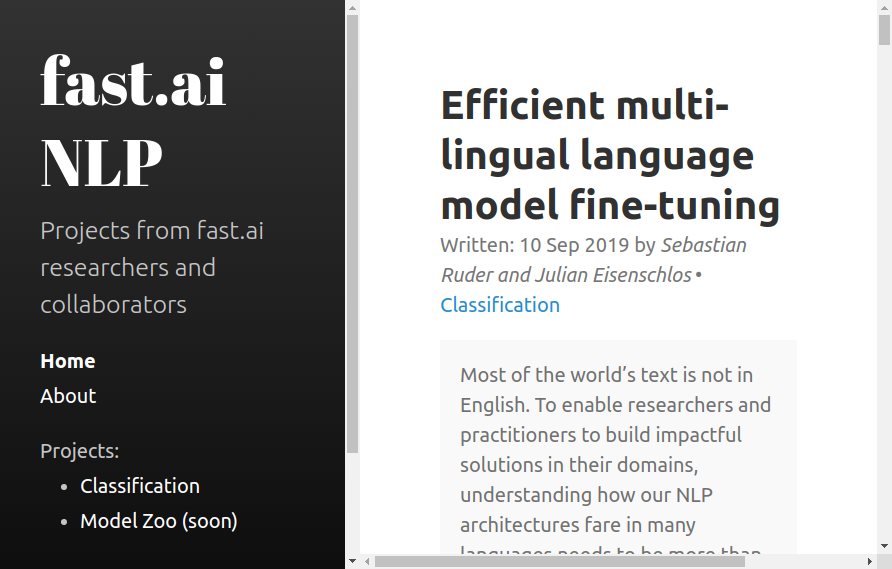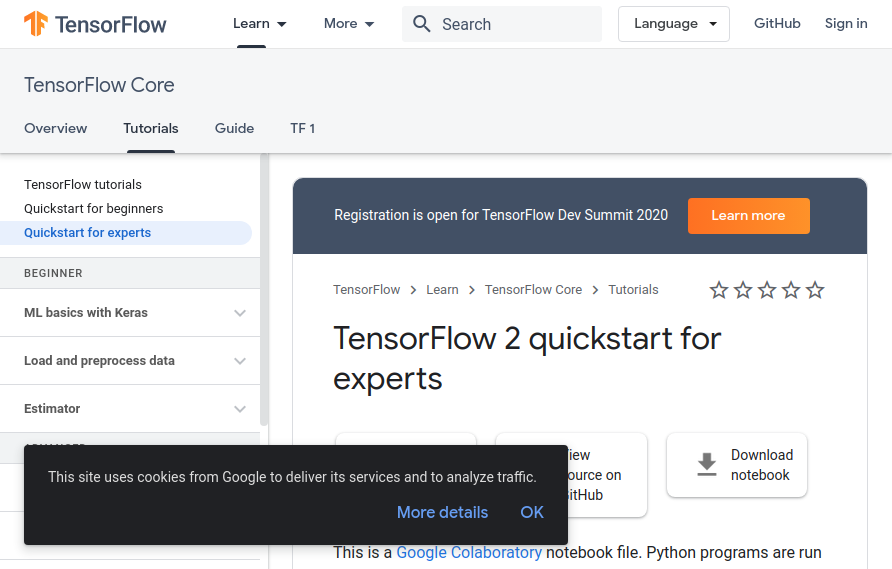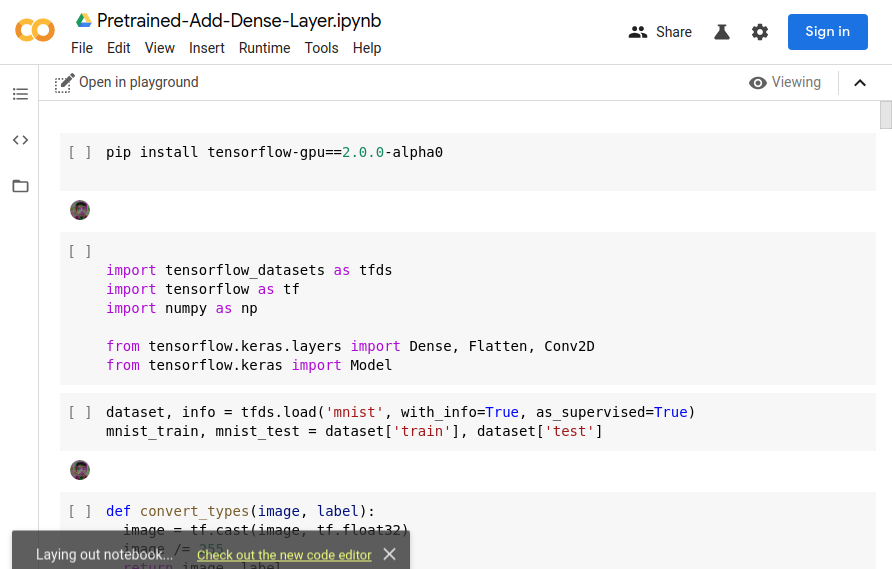Fine-tunning pre-trained neural networks on new data has shown a lot of promise inmanydomains. One simple example is mylast post where I fine-tune OpenAI’s GPT-small model on my chats from facebook messenger to create fake conversation.

Using pre-trained models and further training them is especially useful for organizations with small datasets or resources, and in most cases, it is cost and otherwise effective to do it. However, despite it being widely used, people rarely talk about taking a pre-trained model and making it bigger by adding more layers in the middle of the network rather than just the end.

Naively, this doesn’t work without some tweaks - if you add a layer in the middle of a network then all the trained weights of later layers become useless since they are getting different inputs. There are, however, ways to get around that and I believe that this is an important area to explore as more and more useful models get released.

These posts (along with the last) are my first two in a series where I will attempt to increase the size of OpenAI’s GPT-2 model while taking advantage of the training the model has already gotten. Their model is a great candidate for this experiment, as OpenAI have already demonstrated great results with what is basically a bigger version of it. If anyone else is working on something similar or has links to related research I might have missed - feel free to email me, or hell - even cite me.

Note: The basic network example is mostly taken from theTensorflow 2.0 Getting started article. I used this as an opportunity to play a little with the new API.

You can follow in the colabhere.

## Code

First, we install tensorflow 2.0 to Colab (this step can be skipped after 2.0 is out and the default in Colab)

```pip install tensorflow-gpu==2.0.0-alpha0 ``` Then imports

``````import tensorflow_datasets as tfds
import tensorflow as tf
import numpy as np

from tensorflow.keras.layers import Dense, Flatten, Conv2D
from tensorflow.keras import Model```

Then we download minst to have something to play with, shuffle and batch it

``````

``````dataset, info = tfds.load('mnist', with_info=True, as_supervised=True)
mnist_train, mnist_test = dataset['train'], dataset['test']

def convert_types(image, label):
image = tf.cast(image, tf.float32)
image /= 255
return image, label

mnist_train = mnist_train.map(convert_types).shuffle(10000).batch(32)
mnist_test = mnist_test.map(convert_types).batch(32)
``````

Create our basic model

``````class MyModel(Model):
def __init__(self):
super(MyModel, self).__init__()
self.conv1 = Conv2D(32, 3, activation='relu')
self.flatten = Flatten()
self.d1 = Dense(20, activation='relu')
self.d2 = Dense(10, activation='softmax')

def call(self, x):
x = self.conv1(x)
x = self.flatten(x)
x = self.d1(x)
return self.d2(x)

model = MyModel()
``````

Add our loss, optimizer, and metrics

``````loss_object = tf.keras.losses.SparseCategoricalCrossentropy()

train_loss = tf.keras.metrics.Mean(name='train_loss')
train_accuracy = tf.keras.metrics.SparseCategoricalAccuracy(name='train_accuracy')

test_loss = tf.keras.metrics.Mean(name='test_loss')
test_accuracy = tf.keras.metrics.SparseCategoricalAccuracy(name='test_accuracy')
``````

We define our training_step and test_step functions.

``````@tf.function
def train_step(image, label, model=model):
predictions = model(image)
loss = loss_object(label, predictions)

train_loss(loss)
train_accuracy(label, predictions)
``````
``````@tf.function
def test_step(image, label, model=model):
predictions = model(image)
t_loss = loss_object(label, predictions)

test_loss(t_loss)
test_accuracy(label, predictions)
``````

We can then train our default model a bit.

``````EPOCHS = 2

for epoch in range(EPOCHS):
test_loss.reset_states()
test_accuracy.reset_states()
for image, label in mnist_train:
train_step(image, label)

for test_image, test_label in mnist_test:
test_step(test_image, test_label)

template = 'Epoch {}, Loss: {}, Accuracy: {}, Test Loss: {}, Test Accuracy: {}'
print (template.format(epoch+1,
train_loss.result(),
train_accuracy.result()*100,
test_loss.result(),
test_accuracy.result()*100))
``````

Epoch 1, Loss: 0.20242027938365936, Accuracy: 94.05333709716797, Test Loss: 0.08358743041753769, Test Accuracy: 97.37999725341797

Epoch 2, Loss: 0.13752736151218414, Accuracy: 95.9375, Test Loss: 0.07251566648483276, Test Accuracy: 97.65999603271484

At this point, we can try creating a new bigger model that uses all the weights trained here (including those in the very last layer!).

What we are going to do is make a model almost exactly like the last one but we are going to add one more Dense layer before the final one. The important bit here is to initialize the layer so the weights are in the form of the identity function - this way when the output from the previous layer gets multiplied by the output of this layer we will get exactly the same result, and the weights of the final layer will still make sense. Then during fine-tuning, the layer will slowly move away from the identity function in whichever directions make the most sense. Note: we also want the bias to be initialized to zeros (so we don’t add anything extra to the weights at first) but this is already the default in tensorflow.

``````class MyModel2(Model):
def __init__(self):
super(MyModel2, self).__init__()
self.conv1 = Conv2D(32, 3, activation='relu')
self.flatten = Flatten()
self.d1 = Dense(20, activation='relu')
**self.d_extra = Dense(20, activation='relu', kernel_initializer=tf.keras.initializers.Identity)**
self.d2 = Dense(10, activation='softmax')

def call(self, x):
x = self.conv1(x)
x = self.flatten(x)
x = self.d1(x)
**x = self.d_extra(x)**
return self.d2(x)

model2 = MyModel2()
``````

We then need to re-run our test_step and train_step functions (just re-run the cells containing them) due to how tf.function works. After that, we can confirm our new model isn’t magically performing better than chance.

``````test_loss.reset_states()
test_accuracy.reset_states()
for test_image, test_label in mnist_test:
test_step(test_image, test_label, model2)

template = 'Test Loss: {}, Test Accuracy: {}'

print (template.format(test_loss.result(),
test_accuracy.result()*100))
``````

Test Loss: 2.3064279556274414, Test Accuracy: 10.329999923706055

As expected - it only gets a number right about 1/10th of the time.

Now, the only other thing we need to do is add the weights from our previous model to our new model, except for our new (3rd) layer which will at first just leave things the same.

``````model2.layers.set_weights(model.layers.get_weights())
model2.layers.set_weights(model.layers.get_weights())
model2.layers.set_weights(model.layers.get_weights())
model2.layers.set_weights(model.layers.get_weights())
``````

We can then re-run the code checking the accuracy of our new model and voila

Test Loss: 0.07251566648483276, Test Accuracy: 97.65999603271484

At this point we can just start training our bigger model.

``````EPOCHS = 2

for epoch in range(EPOCHS):
test_loss.reset_states()
test_accuracy.reset_states()
for image, label in mnist_train:
train_step(image, label, model2)

for test_image, test_label in mnist_test:
test_step(test_image, test_label, model2)

template = 'Epoch {}, Loss: {}, Accuracy: {}, Test Loss: {}, Test Accuracy: {}'
print (template.format(epoch+1,
train_loss.result(),
train_accuracy.result()*100,
test_loss.result(),
test_accuracy.result()*100))
``````

Which gets me to

Epoch 1, Loss: 0.11430724710226059, Accuracy: 96.58721923828125, Test Loss: 0.05486641451716423, Test Accuracy: 98.18999481201172

Epoch 2, Loss: 0.09389258921146393, Accuracy: 97.19083404541016, Test Loss: 0.05310175567865372, Test Accuracy: 98.31999969482422

Not much better, but the results will usually be more impressive when dealing with more complex problems.

Also, instead of doing that, we can also freeze everything but our new layer to more accurately only train it. This will train faster, and depending on the problem, it might make more sense - or it might make more sense for a few epochs before again training all layers. Play around with it!

``````@tf.function
def train_step(image, label, model=model):
predictions = model(image)
loss = loss_object(label, predictions)

train_loss(loss)
train_accuracy(label, predictions)
``````

The way we do that is by using the gradients of only our new layer. model.trainable_variables works by returning a list of all weights and biases of our model layer by layer - so the first item is the weights of layer 1, 2nd item is the bias of layer 1 etc. Thus we only need the 2 layers before the last 2.

## Fully convolutional

What if we are working with e.g. a fully convolutional network - an identity matrix won’t work (and tensorflow doesn’t even allow us to use the identity initializer for that reason) - how do you add a new layer while keeping the network usable?

There are different ways you can extend the network while keeping all the weights useful - one I like is to use a residual-like approach. I simply initialize the new conv layer using all 1s and multiply the output from it to the output of the previous layer. This changes nothing at first but once we start training again, we can slowly move those weights in the direction we want them to be.

``````class MyModel(Model):
def __init__(self):
super(MyModel, self).__init__()
self.conv1 = Conv2D(32, 3, activation='relu', padding='SAME')
self.flatten = Flatten()
self.d1 = Dense(10, activation='softmax')

def call(self, x):
x = self.conv1(x)
x = self.flatten(x)
return self.d1(x)

class MyModel2(Model):
def __init__(self):
super(MyModel2, self).__init__()
self.conv1 = Conv2D(32, 3, activation='relu', padding='SAME')
self.c_extra = Conv2D(32, 3, activation='relu', padding='SAME', kernel_initializer=tf.keras.initializers.Ones)
self.flatten = Flatten()
self.d1 = Dense(10, activation='softmax')

def call(self, x):
x = self.conv1(x)
x = x * self.c_extra(x)
x = self.flatten(x)
return self.d1(x)
``````

The rest is exactly the same as before.

## Conclusion

Increasing the size of a network is something usually done before training from scratch but that doesn’t always need to be the case. You can add one or better yet more layers across the network or even at the very start. Whether this will help depends - mainly on whether you needed a bigger net from the start - but it can definitely save you time.